# HDOJ 5418-Victor and World

HDOJ 5418-Victor and World
Victor and World
Time Limit: 4000/2000 MS (Java/Others) Memory Limit: 262144/131072 K (Java/Others)
Total Submission(s): 643 Accepted Submission(s): 268
Problem Description
After trying hard for many years, Victor has finally received a pilot license. To have a celebration, he intends to buy himself an airplane and fly around the world. There are n countries on the earth, which are numbered from 1 to n. They are connected by m undirected flights, detailedly the i-th flight connects the ui-th and the vi-th country, and it will cost Victor's airplane wi L fuel if Victor flies through it. And it is possible for him to fly to every country from the first country.
Victor now is at the country whose number is 1, he wants to know the minimal amount of fuel for him to visit every country at least once and finally return to the first country.
Input
The first line of the input contains an integer T, denoting the number of test cases.
In every test case, there are two integers n and m in the first line, denoting the number of the countries and the number of the flights.
Then there are m lines, each line contains three integers ui, vi and wi, describing a flight.
1≤T≤20.
1≤n≤16.
1≤m≤100000.
1≤wi≤100.
1≤ui,vi≤n.
Output
Your program should print T lines : the i-th of these should contain a single integer, denoting the minimal amount of fuel for Victor to finish the travel.
Sample Input
1
3 2
1 2 2
1 3 3
Sample Output
10
Source
BestCoder Round #52 (div.2)
Recommend
hujie | We have carefully selected several similar problems for you: 5421 5420 5419 5416 5415

$dp[s|(1<

#include<iostream>
#include<cstdio>
#include<cstring>
#include<algorithm>
using namespace std;
const int kMaxN = 17, kINF = 0x3f3f3f3f;
int dis[kMaxN][kMaxN], dp[1 << kMaxN][kMaxN];
int n, m;
void Init()
{
memset(dis, 0x3f, sizeof(dis));
memset(dp, 0x3f, sizeof(dp));
for (int i = 1; i <= n; i++)
dis[i][i] = 0;
}
void Floyd()
{
for (int k = 1; k <= n; k++)
for (int i = 1; i <= n; i++)
for (int j = 1; j <= n; j++)
dis[i][j] = min(dis[i][k] + dis[k][j], dis[i][j]);
}
int main()
{
freopen("input.txt", "r", stdin);
int t, u, v, w;
scanf("%d", &t);
while (t--)
{
scanf("%d %d", &n, &m);
Init();
while (m--)
{
scanf("%d %d %d", &u, &v, &w);
dis[u][v] = min(w, dis[u][v]);
dis[v][u] = dis[u][v];
}
Floyd();
dp = 0;
for (int status = 1; status < (1 << n); status++)//枚举状态
{
for (int i = 1; i <= n; i++)//枚举最后访问的城市
{
//if (status&(1 << (i-1))==0)//(1)
if ((status&(1 << (i-1)))==0)//(2)i有没有访问过都可以
{
for (int j = 1; j <= n; j++)//枚举中间转折点
{
if (status&(1 << (j-1)))//(3)转折点j一定要访问过
dp[status | (1 << (i-1))][i] = min(dp[status | (1 << (i-1))][i], dp[status][j] + dis[i][j]);
}
}
}
}
int ans = kINF;
for (int i = 1; i <= n; i++)
ans = min(ans, dp[(1 << n) - 1][i] + dis[i]);
printf("%d\n", n == 1 ? 0 : ans);
}
return 0;
}


# POJ 1094-Sorting It All Out

POJ 1094-Sorting It All Out
Sorting It All Out
Time Limit: 1000MS Memory Limit: 10000K
Total Submissions: 28397 Accepted: 9814
Description
An ascending sorted sequence of distinct values is one in which some form of a less-than operator is used to order the elements from smallest to largest. For example, the sorted sequence A, B, C, D implies that A < B, B < C and C < D. in this problem, we will give you a set of relations of the form A < B and ask you to determine whether a sorted order has been specified or not.
Input
Input consists of multiple problem instances. Each instance starts with a line containing two positive integers n and m. the first value indicated the number of objects to sort, where 2 <= n <= 26. The objects to be sorted will be the first n characters of the uppercase alphabet. The second value m indicates the number of relations of the form A < B which will be given in this problem instance. Next will be m lines, each containing one such relation consisting of three characters: an uppercase letter, the character "<" and a second uppercase letter. No letter will be outside the range of the first n letters of the alphabet. Values of n = m = 0 indicate end of input.
Output
For each problem instance, output consists of one line. This line should be one of the following three:
Sorted sequence determined after xxx relations: yyy...y.
Sorted sequence cannot be determined.
Inconsistency found after xxx relations.
where xxx is the number of relations processed at the time either a sorted sequence is determined or an inconsistency is found, whichever comes first, and yyy...y is the sorted, ascending sequence.
Sample Input
4 6
A<B
A<C
B<C
C<D
B<D
A<B
3 2
A<B
B<A
26 1
A<Z
0 0
Sample Output
Sorted sequence determined after 4 relations: ABCD.
Inconsistency found after 2 relations.
Sorted sequence cannot be determined.
Source
East Central North America 2001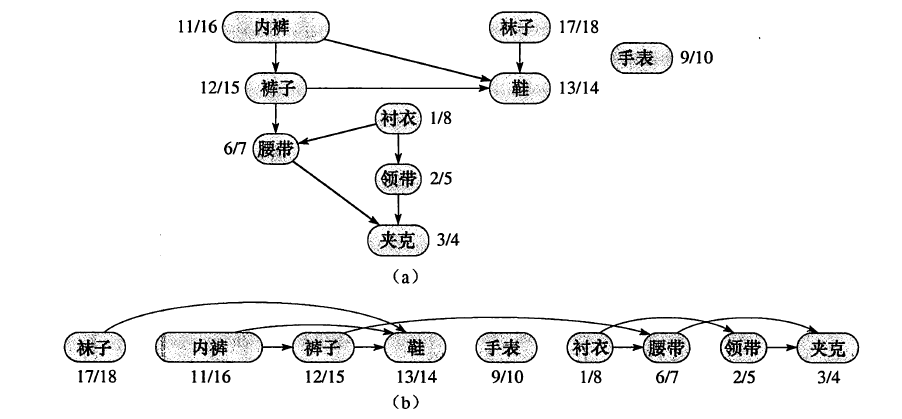Kahn算法

L ← Empty list that will contain the sorted elements
S ← Set of all nodes with no incoming edges
while S is non-empty do
remove a node n from S
add n to tail of L
for each node m with an edge e from n to m do
remove edge e from the graph
if m has no other incoming edges then
insert m into S
if graph has edges then
return error (graph has at least one cycle)
else
return L (a topologically sorted order)


L ← Empty list that will contain the sorted nodes
while there are unmarked nodes do
select an unmarked node n
visit(n)
function visit(node n)
if n has a temporary mark then stop (not a DAG)
if n is not marked (i.e. has not been visited yet) then
mark n temporarily
for each node m with an edge from n to m do
visit(m)
mark n permanently
unmark n temporarily
add n to head of L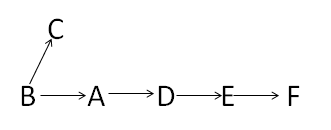#include<iostream>
//#include<set>
#include<list>
#include<string>
#include<vector>
using namespace std;
int n,m;
const int MAX_N=26;
const int MAX_DIS=10000;
//*******这些都是维基百科关于拓扑排序（DFS版）里的变量含义
int temporary[MAX_N];
int permanent[MAX_N];
int marked[MAX_N];
//*******************************
int path[MAX_N][MAX_N];
//int dfs_visited[MAX_N];
list<int> L;//拓扑排序生产的顺序链
bool isDAG;//DAG=directed acyclic graph，无回路有向图
//每一个测试用例都要初始化路径
void init_path()
{
for(int i=0;i<n;i++)
for(int j=0;j<n;j++)
path[i][j]=0;
}
//每一次拓扑排序都要初始化temporary，permanent，marked
void init_tpm()
{
isDAG=true;
L.clear();
for(int i=0;i<n;i++)
{
temporary[i]=0;
permanent[i]=0;
marked[i]=0;
}
}
//递归访问。具体看维基百科
void visit(int i)
{
if(temporary[i]==1)
{
isDAG=false;
return;
}
else
{
if(marked[i]==0)
{
marked[i]=1;
temporary[i]=1;
for(int j=0;j<n;j++)
{
if(path[i][j]==1)
{
visit(j);
}
}
permanent[i]=1;
temporary[i]=0;
L.push_front(i);
}
}
}
/*
void init_dfs()
{
for(int i=0;i<n;i++)
dfs_visited[i]=0;
}*/
/*
//DFS有缺陷
void DFS(int v)
{
if(dfs_visited[v]==0)
{
dfs_visited[v]=1;
for(int i=0;i<n;i++)
{
if(dfs_visited[i]==0&&path[v][i]==1)
{
DFS(i);
}
}
}
}*/
//使用Floyd算法来判断生产的拓扑排序是否是严格有序的
bool Floyd()
{
int copy_path[MAX_N][MAX_N];
for(int i=0;i<n;i++)//首先复制一份路径图
for(int j=0;j<n;j++)
{
copy_path[i][j]=path[i][j];
if(i!=j&&copy_path[i][j]==0)//如果原来两点距离为0，说明他们是不直接连通的
copy_path[i][j]=MAX_DIS;//置为无穷
}
//floyd算法
for(int k=0;k<n;k++)
for(int i=0;i<n;i++)
for(int j=0;j<n;j++) if(copy_path[i][j]>copy_path[i][k]+copy_path[k][j])
copy_path[i][j]=copy_path[i][k]+copy_path[k][j];
vector<int> seq;//把原来用链表的拓扑序列转换成数组，方便后面的操作
list<int>::iterator it=L.begin();
while(it!=L.end())
{
seq.push_back(*it);
it++;
}
//如果这个拓扑链是严格有序的话，则前面的元素到后面的元素一定是可达的。
for(int i=0;i<n-1;i++)
{
for(int j=i+1;j<n;j++) { if(copy_path[seq[i]][seq[j]]>=MAX_DIS)//如果不可达，则不是严格有序的。
return false;
}
}
return true;
}
//拓扑排序DFS版本。返回0：有回路；1：虽然是拓扑排序，但非连通（不是严格有序）；2：是拓扑排序且连通（严格有序）（即任意两个元素都可以比较大小）
int topological_sorting()
{
for(int i=0;i<n;i++)
{
if(marked[i]==0)
{
visit(i);
}
}
if(!isDAG)
return 0;
else
{
/*init_dfs();
DFS(*L.begin());
for(int i=0;i<n;i++) { if(dfs_visited[i]==0) { return 1; } }*/ if(Floyd()) return 2; else return 1; } } int main() { //freopen("input.txt","r",stdin); string tmp; while(cin>>n>>m&&n&&m)
{
init_path();
vector<string> relations(m);
int i;
for(i=0;i<m;i++)//一次性把所有输入都存起来，免得后续麻烦 { cin>>relations[i];
}
int rs=-1;
for(i=0;i<m;i++)//每增加一个关系，都要重新拓扑排序一次
{
init_tpm();//每次都要初始化
tmp=relations[i];
path[tmp-'A'][tmp-'A']=1;
rs=topological_sorting();
if(rs==0)
{
cout<<"Inconsistency found after "<<i+1<<" relations."<<endl;
break;//如果是回路的话，后续的输入可以跳过
}
else if(rs==2)//成功
{
cout<<"Sorted sequence determined after "<<i+1<<" relations: ";
list<int>::iterator it=L.begin();
while(it!=L.end())
{
char c='A'+*it;
cout<<c;
it++;
}
cout<<"."<<endl;
break;//后续输入跳过
}
}
if(i==m&&rs==1)//如果处理完所有输入都没有形成严格有序的拓扑序列
cout<<"Sorted sequence cannot be determined."<<endl;
}
return 0;
}


# POJ 2263-Heavy Cargo

POJ 2263-Heavy Cargo
Heavy Cargo
Time Limit: 1000MS Memory Limit: 65536K
Total Submissions: 3549 Accepted: 1894
Description
Big Johnsson Trucks Inc. is a company specialized in manufacturing big trucks. Their latest model, the Godzilla V12, is so big that the amount of cargo you can transport with it is never limited by the truck itself. It is only limited by the weight restrictions that apply for the roads along the path you want to drive.
Given start and destination city, your job is to determine the maximum load of the Godzilla V12 so that there still exists a path between the two specified cities.
Input
The input will contain one or more test cases. The first line of each test case will contain two integers: the number of cities n (2<=n<=200) and the number of road segments r (1<=r<=19900) making up the street network.
Then r lines will follow, each one describing one road segment by naming the two cities connected by the segment and giving the weight limit for trucks that use this segment. Names are not longer than 30 characters and do not contain white-space characters. Weight limits are integers in the range 0 - 10000. Roads can always be travelled in both directions.
The last line of the test case contains two city names: start and destination.
Input will be terminated by two values of 0 for n and r.
Output
For each test case, print three lines:
a line saying "Scenario #x" where x is the number of the test case
a line saying "y tons" where y is the maximum possible load
a blank line
Sample Input
4 3
Karlsruhe Stuttgart 100
Stuttgart Ulm 80
Ulm Muenchen 120
Karlsruhe Muenchen
5 5
Karlsruhe Stuttgart 100
Stuttgart Ulm 80
Ulm Muenchen 120
Karlsruhe Hamburg 220
Hamburg Muenchen 170
Muenchen Karlsruhe
0 0
Sample Output
Scenario #1
80 tons
Scenario #2
170 tons
Source
Ulm Local 1998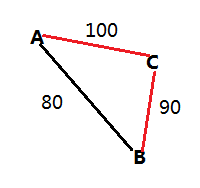#include<iostream>
#include<map>
#include<string>
using namespace std;
const int MAX_N=202;
int load_ton[MAX_N][MAX_N];
//初始化数据
void init_load(int n)
{
for(int i=0;i<n;i++)
for(int j=0;j<n;j++)
load_ton[i][j]=0;
}
//改造Floyd算法
void Floyd(int n)
{
for(int k=0;k<n;k++)
{
for(int i=0;i<n;i++)
{
for(int j=0;j<n;j++)
{
int min_ton=load_ton[i][k]<load_ton[k][j]?load_ton[i][k]:load_ton[k][j];//这条路上的最小值即为能通过的最大值
if(load_ton[i][j]<min_ton)
{
load_ton[i][j]=min_ton;//对称矩阵
load_ton[j][i]=min_ton;//对称矩阵
}
}
}
}
}
int main()
{
//freopen("input.txt","r",stdin);
int n,r;
int case_num=1;
while(cin>>n>>r&&n&&r)
{
init_load(n);
map<string,int> city_index;//保存城市和下标的对应关系
string city1,city2;
int w;
int i=0;
while(r--)
{
cin>>city1>>city2>>w;
if(city_index.find(city1)==city_index.end())
city_index[city1]=i++;
if(city_index.find(city2)==city_index.end())
city_index[city2]=i++;
//load_ton[i-2][i-1]=w;//错，因为有可能cityi在之前已经加入了，这个时候i-1,i-2就不是cityi的下标了
//load_ton[i-1][i-2]=w;
load_ton[city_index[city1]][city_index[city2]]=w;//还是要从map里找对应下标
load_ton[city_index[city2]][city_index[city1]]=w;//因为路是两边通的，所以a[i][j]和a[j][i]相等
}
cin>>city1>>city2;
Floyd(n);
cout<<"Scenario #"<<case_num++<<endl;//
cout<<load_ton[city_index[city1]][city_index[city2]]<<" tons"<<endl<<endl;
}
return 0;
}


# POJ 2240-Arbitrage

POJ 2240-Arbitrage
Arbitrage
Time Limit: 1000MS Memory Limit: 65536K
Total Submissions: 16195 Accepted: 6814
Description
Arbitrage is the use of discrepancies in currency exchange rates to transform one unit of a currency into more than one unit of the same currency. For example, suppose that 1 US Dollar buys 0.5 British pound, 1 British pound buys 10.0 French francs, and 1 French franc buys 0.21 US dollar. Then, by converting currencies, a clever trader can start with 1 US dollar and buy 0.5 * 10.0 * 0.21 = 1.05 US dollars, making a profit of 5 percent.
Your job is to write a program that takes a list of currency exchange rates as input and then determines whether arbitrage is possible or not.
Input
The input will contain one or more test cases. Om the first line of each test case there is an integer n (1<=n<=30), representing the number of different currencies. The next n lines each contain the name of one currency. Within a name no spaces will appear. The next line contains one integer m, representing the length of the table to follow. The last m lines each contain the name ci of a source currency, a real number rij which represents the exchange rate from ci to cj and a name cj of the destination currency. Exchanges which do not appear in the table are impossible.
Test cases are separated from each other by a blank line. Input is terminated by a value of zero (0) for n.
Output
For each test case, print one line telling whether arbitrage is possible or not in the format "Case case: Yes" respectively "Case case: No".
Sample Input
3
USDollar
BritishPound
FrenchFranc
3
USDollar 0.5 BritishPound
BritishPound 10.0 FrenchFranc
FrenchFranc 0.21 USDollar
3
USDollar
BritishPound
FrenchFranc
6
USDollar 0.5 BritishPound
USDollar 4.9 FrenchFranc
BritishPound 10.0 FrenchFranc
BritishPound 1.99 USDollar
FrenchFranc 0.09 BritishPound
FrenchFranc 0.19 USDollar
Sample Output
Case 1: Yes
Case 2: No
Source
Ulm Local 1996

#include<iostream>
#include<string>
#include<map>
using namespace std;
const int MAX_N=31;
double path[MAX_N][MAX_N];
//初始化，A到A的路径（汇率）为1；其他为0
void init_path(int n)
{
for(int i=0;i<n;i++)
{
for(int j=0;j<n;j++)
{
if(i==j)
path[i][j]=1.0;
else
path[i][j]=0.0;
}
}
}
//Floyd算法求所有点对的“最短距离”（最大乘积值）
void Floyd(int n)
{
for(int k=0;k<n;k++)
{
for(int i=0;i<n;i++)
{
for(int j=0;j<n;j++)
{
path[i][j]=(path[i][j]>path[i][k]*path[k][j])?path[i][j]:(path[i][k]*path[k][j]);
}
}
}
}
int main()
{
//freopen("input.txt","r",stdin);
int n,m;
int case_num=1;
while(cin>>n&&n)
{
init_path(n);
map<string,int> currency_index;//保存string和int的对应关系
int i=0;
string tmp;
for(int j=0;j<n;j++)
{
cin>>tmp;
currency_index[tmp]=i++;
}
cin>>m;
string ci,cj;
double rij;
while(m--)
{
cin>>ci>>rij>>cj;
path[currency_index[ci]][currency_index[cj]]=rij;
}
Floyd(n);
bool yes=false;
for(i=0;i<n;i++)
{
if(path[i][i]>1)
{
yes=true;
break;
}
}
if(yes)
cout<<"Case "<<case_num<<": Yes"<<endl;
else
cout<<"Case "<<case_num<<": No"<<endl;
case_num++;
}
return 0;
}


# POJ 1125-Stockbroker Grapevine

POJ 1125-Stockbroker Grapevine
Stockbroker Grapevine
Time Limit: 1000MS Memory Limit: 10000K
Total Submissions: 27559 Accepted: 15273
Description
Stockbrokers are known to overreact to rumours. You have been contracted to develop a method of spreading disinformation amongst the stockbrokers to give your employer the tactical edge in the stock market. For maximum effect, you have to spread the rumours in the fastest possible way.
Unfortunately for you, stockbrokers only trust information coming from their "Trusted sources" This means you have to take into account the structure of their contacts when starting a rumour. It takes a certain amount of time for a specific stockbroker to pass the rumour on to each of his colleagues. Your task will be to write a program that tells you which stockbroker to choose as your starting point for the rumour, as well as the time it will take for the rumour to spread throughout the stockbroker community. This duration is measured as the time needed for the last person to receive the information.
Input
Your program will input data for different sets of stockbrokers. Each set starts with a line with the number of stockbrokers. Following this is a line for each stockbroker which contains the number of people who they have contact with, who these people are, and the time taken for them to pass the message to each person. The format of each stockbroker line is as follows: The line starts with the number of contacts (n), followed by n pairs of integers, one pair for each contact. Each pair lists first a number referring to the contact (e.g. a '1' means person number one in the set), followed by the time in minutes taken to pass a message to that person. There are no special punctuation symbols or spacing rules.
Each person is numbered 1 through to the number of stockbrokers. The time taken to pass the message on will be between 1 and 10 minutes (inclusive), and the number of contacts will range between 0 and one less than the number of stockbrokers. The number of stockbrokers will range from 1 to 100. The input is terminated by a set of stockbrokers containing 0 (zero) people.
Output
For each set of data, your program must output a single line containing the person who results in the fastest message transmission, and how long before the last person will receive any given message after you give it to this person, measured in integer minutes.
It is possible that your program will receive a network of connections that excludes some persons, i.e. some people may be unreachable. If your program detects such a broken network, simply output the message "disjoint". Note that the time taken to pass the message from person A to person B is not necessarily the same as the time taken to pass it from B to A, if such transmission is possible at all.
Sample Input
3
2 2 4 3 5
2 1 2 3 6
2 1 2 2 2
5
3 4 4 2 8 5 3
1 5 8
4 1 6 4 10 2 7 5 2
2 2 5 1 5
Sample Output
3 2
3 10
Source
Southern African 2001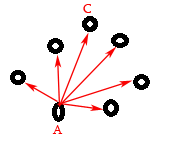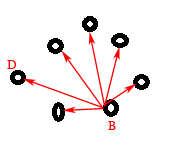1. 测试图中的每一个点$i$，求从该点出发，到其他所有点的单源最短距离集合$S_i$
2. 求出该单源最短距离$S_i$内部的最大值$M_i$
3. 对于图中的所有点，求其$M_i$
4. 最后求所有$M_i$中的最小值$MIN$，即为最终结果

#include<iostream>
using namespace std;
const int MAX_DISTANCE=1024;//距离取值范围[1,10]，所以用1024代表无穷大
const int MAX_PEOPLE_NUM=101;//最多传播者
int path[MAX_PEOPLE_NUM][MAX_PEOPLE_NUM];//路径
int n;//实际人数
//初始化路径都为0
void init_path()
{
for(int i=0;i<n;i++)
for(int j=0;j<n;j++)
path[i][j]=0;
}
//floyd算法求所有点对的最短距离
void floyd()
{
for(int k=0;k<n;k++)
{
for(int i=0;i<n;i++)
{
for(int j=0;j<n;j++)
{
if(path[i][j]>path[i][k]+path[k][j])
path[i][j]=path[i][k]+path[k][j];
}
}
}
}
int main()
{
int connected_num,person_index;
while(cin>>n&&n)
{
init_path();
//输入数据
for(int i=0;i<n;i++)
{
cin>>connected_num;
for(int j=0;j<connected_num;j++)
{
cin>>person_index;
cin>>path[i][person_index-1];
}
}
//给不相连的两者的距离赋为最大值无穷
for(int i=0;i<n;i++)
{
for(int j=0;j<n;j++)
{
if(i!=j&&path[i][j]==0)
path[i][j]=MAX_DISTANCE;
}
}
floyd();//调用floyd算法，求得所有点对的最短距离，还是保存在path[][]中
int longest_path,global_shorest=MAX_DISTANCE,start_person;
for(int i=0;i<n;i++)//对于以i为起点的单源最短距离
{
longest_path=0;//求出这个单源最短距离中的最大值
for(int j=0;j<n;j++)
if(path[i][j]>longest_path)
longest_path=path[i][j];
if(longest_path<global_shorest)//再求其最小值
{
start_person=i;
global_shorest=longest_path;
}
}
if(global_shorest<MAX_DISTANCE)
cout<<start_person+1<<" "<<global_shorest<<endl;
else
cout<<"disjoint"<<endl;//非连通图
}
return 0;
}